Latest Banking jobs   »

# Reasoning Ability Quiz For Bank Foundation 2023-16th February

Direction (1-3): Read the following information carefully and answer the questions given below.
In a family of three-generation, there are eight members i.e. A, E, H, N, K, L, G and D. A is the sister-in-law of D who has no sibling. N is the parent of G’s sibling. L who is not the male member of the family is not the sibling of G’s mother. H is the father of E who is the only nephew of A. A’s mother is the only sibling of G’s mother.

Q1. What is the relation of A’s sister-in-law with E?
(a) Mother
(b) Maternal Aunt
(c) Paternal Uncle
(d) Sister
(e) None of these

Q2. If L’s sibling is the son of P then what is the relation of P with respect to K?
(a) Father
(b) Brother-in-law
(c) Sister-in-law
(d) Uncle
(e) None of these

Q3. How many male members are there in the family?
(a) Two
(b) Four
(c) Three
(d) More than four
(e) Can’t be determined

Direction (4-6): Read the following information carefully and answer the questions given below.
A family consists of eight persons out of which three are married couples. F is N’s son and B is M’s son. Z is not a child of R. F is R’s brother-in-law. D is Z’s mother and Y’s aunt. B is the uncle of Z and husband of R.

Q4. Which is not a married couple out of the given options?
(a) D and F
(b) B and R
(c) N and M
(d) Y and Z
(e) Cannot be determined

Q5. Who is N’s grandson?
(a) Y
(b) B
(c) F
(d) Z
(e) Cannot be determined

Q6. Who are the daughters in law of M?
(a) R and B
(b) F and D
(c) R and D
(d) Y and N
(e) Z and N

Direction (7-9): Study the following information carefully and answer the following questions.
There are eight people in a family with two married couples. B is the brother-in-law of A. R is the mother of J. U is not the female. K is the sister of A. Z is the grandfather of U and V. K has only one son and one daughter. Z is the father of A who is married to R.

Q7. How J is related to Z?
(a) Grandson
(b) Grand-daughter
(c) Daughter
(d) Son
(e) Can’t be determined

Q8. Who is the son of B?
(a) A
(b) J
(c) V
(d) U
(e) None of these

Q9. Find the odd one.
(a) U
(b) J
(c) A
(d) R
(e) V

Direction (10-12): Study the following information carefully and answer the following questions.
In a three-generation family of nine members i.e., K, L, M, N, O, P, Q, R and S there are two married couples. K is the spouse of O who is the sister-in-law of S. M is the sister of N who is the mother of Q. R is the brother of Q. Q is the granddaughter of P who is the brother of O. L is the brother-in-law of M. P is not parent of L.

Q10. What is the relation of P with respect to L?
(a) Father- in- law
(b) Brother
(c) Father
(d) Grandfather
(e) None of these

Q11. Who amongst the following is the father of Q?
(a) M
(b) L
(c) K
(d) S
(e) None of these

Q12. What is the relation of Q with respect to M?
(a) Grand-son
(b) Niece
(c) Daughter
(d) Son
(e) None of these

Direction (13-15): Study the following information carefully and answer the following questions.
In a three-generation family of 11 members, B is the sister of C and D. Q is the father of A who is sibling of B. J has only two sons and H has only one daughter. M is the daughter of D. T and N are grandsons of P. D is uncle of T. J is a female and married to C. A has no child.

Q13. Who is the mother of B?
(a) J
(b) P
(c) H
(d) A
(e) None of these

Q14. How is N related to J?
(a) Father
(b) Nephew
(c) Son
(d) Brother
(e) None of these

Q15. How many female members are there in the family?
(a) Three
(b) Four
(c) More than five
(d) Five
(e) Can’t be determined

Solutions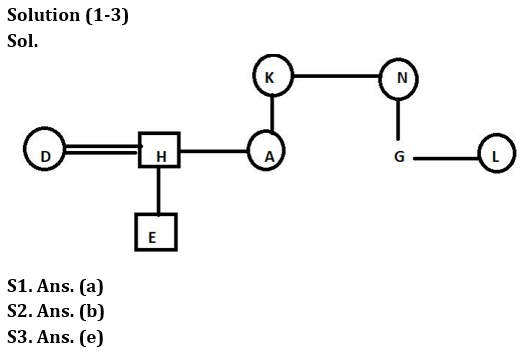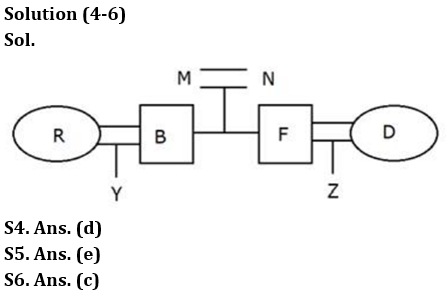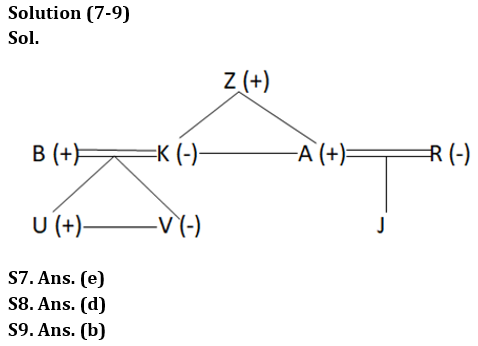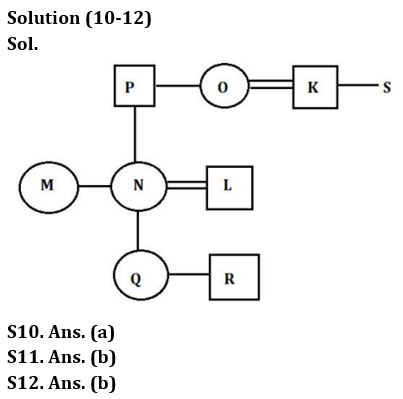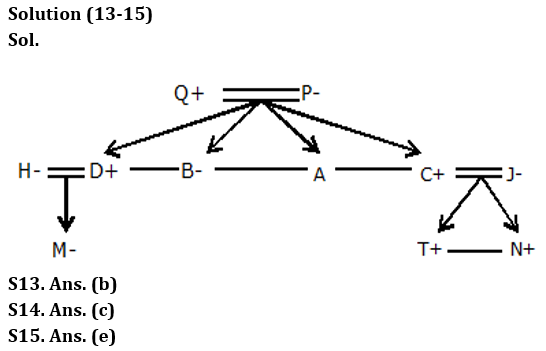## FAQs

### What is the selection process of the Bank Clerk?

The selection process of the Bank Clerk is Prelims & Mains.

#### Congratulations!Union Budget 2023-24: Free PDF